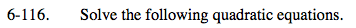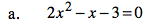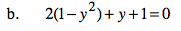### Home > PC > Chapter 6 > Lesson 6.3.1 > Problem6-116

6-116.
1. Solve the following quadratic equations. Homework Help ✎

1. 2x2x − 3 = 0

2. 2(1 − y2) + y + 1 = 0Factor setting each factor = to 0.1. Distribute.
2. Simplify.
3. Factor setting each factor = to 0.
4. What do you notice about parts (a) and (b)?